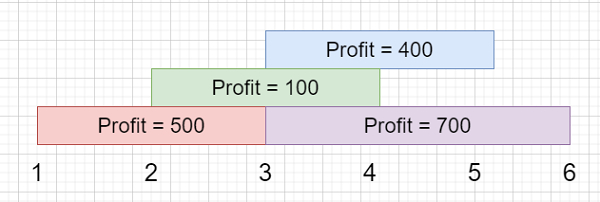# Maximum Profit in Job Scheduling in C++

Suppose we have n different tasks, where every task is scheduled to be done from startTime[i] to endTime[i], for that task we algo get profit of profit[i]. We know the startTime , endTime and profit lists, we have to find the maximum profit we can take such that there are no 2 tasks in the subset with overlapping time range. If we choose a task that ends at time X we will be able to start another task that starts at time X.

So, if the input is like startTime = [1,2,3,3], endTime = [3,4,5,6] profit = [500,100,400,700]then the output will be 1200

To solve this, we will follow these steps −

• Define one Data with start, end and cost values
• make one array of Data j

• n := size of s

• for initialize i := 0, when i < n, update (increase i by 1), do −

• Create one Data temp(s[i], e[i], p[i])

• insert temp at the end of j

• sort the array j based on the end time

• Define an array dp of size n

• dp := j.cost

• for initialize i := 1, when i < n, update (increase i by 1), do −

• temp := 0, low := 0, high := i - 1

• while low < high, do −

• mid := low + (high - low + 1) / 2

• if j[mid].end <= j[i].start, then −

• low := mid

• Otherwise

• high := mid - 1

• dp[i] := j[i].cost

• if j[low].end <= j[i].start, then −

• dp[i] := dp[i] + dp[low]

• dp[i] := maximum of dp[i] and dp[i - 1]

• return dp[n - 1]

Let us see the following implementation to get better understanding −

## Example

Live Demo

#include <bits/stdc++.h>
using namespace std;
struct Data{
int s,e,c;
Data(int x, int y, int z){
s= x;
e= y;
c = z;
}
};
bool cmp(Data a, Data b){
return a.e<b.e;
}
class Solution {
public:
int jobScheduling(vector<int>& s, vector<int>& e, vector<int>& p){
vector<Data> j;
int n = s.size();
for (int i = 0; i < n; i++) {
Data temp(s[i], e[i], p[i]);
j.push_back(temp);
}
sort(j.begin(), j.end(), cmp);
vector<int> dp(n);
dp = j.c;
for (int i = 1; i < n; i++) {
int temp = 0;
int low = 0;
int high = i - 1;
while (low < high) {
int mid = low + (high - low + 1) / 2;
if (j[mid].e <= j[i].s)
low = mid;
else
high = mid - 1;
}
dp[i] = j[i].c;
if (j[low].e <= j[i].s)
dp[i] += dp[low];
dp[i] = max(dp[i], dp[i - 1]);
}
return dp[n - 1];
}
};
main(){
Solution ob;
vector<int> startTime = {1,2,3,3}, endTime = {3,4,5,6}, profit =
{500,100,400,700};
cout << (ob.jobScheduling(startTime, endTime, profit));
}

## Input

{1,2,3,3}, {3,4,5,6}, {500,100,400,700}

## Output

1200